﻿ Standard model is wrong, Quark isn't real.

# Standard model is wrong. Quark isn't real.

## Unreal "Symmetry" is just math, NOT physics.

I congratulate Englert and Higgs on winning Nobel physics prize.
But sorry for them, we have to look at again the present chaotic situation of physics calmly. Not neglect fatal paradoxes.
It is unfortunate that we're making no progress, "Shut up and calculate !" physics continues from now on...

(Fig.1) "Symmetry" is just mathematical symbols. NOT physics.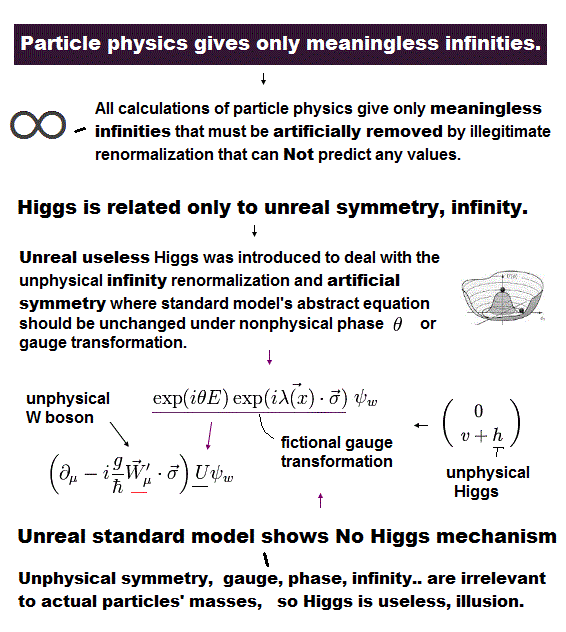We often hear the news that they have found God particle "Higgs", the last piece of the puzzle.
So standard model becomes complete, and proves to be right.
But if standard model is correct, the progresses in physics and all other fields stop, and things would be much worse.

As shown on this page, the present standard model completely depend on "mathematical" symmetry.
Unfortunately these symmetries are ONLY "math" symbols, and have NO physical entity at all.
They just play with abstract "math" and symbols ( NOT aiming at reality forever ).

## Standard model is correct → ONLY 10-12 dimensional string ( M, F ) theory is left.

(Fig.2) Standard model is correct → ONLY unrealistic string theory and many worlds are left !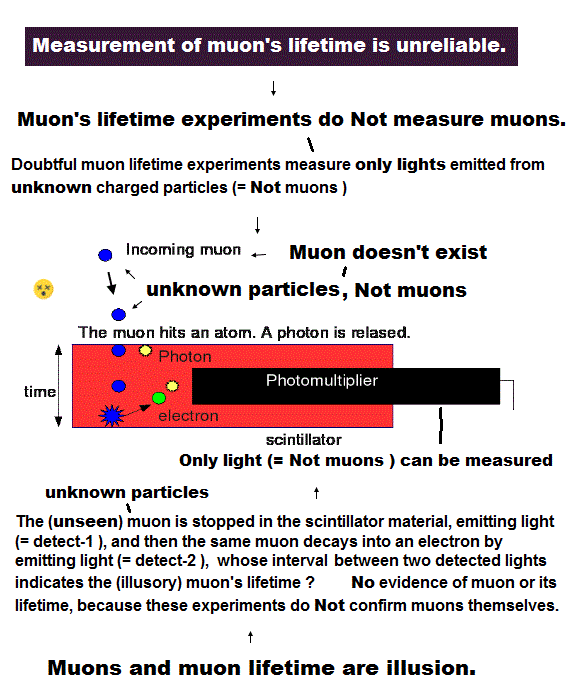As shown on this page, after 40 years, ONLY unrealistic 10 dimentional string theory is left as unified theory.
( Loop quantum gravity completely failed as unified theory due to its unreal concepts about space. )

So if you accept this standard model, you MUST believe unrealistic another dimensions, though they are just "fictions".
In addition to these fictional dimensions, string theory, very abstract math symbols, clearly obstructs the development of actual physics, and destroys many superior talents of young students.

Furthermore, all you can do is to accept unrealistic many-world superposition, faster-than-light inflation, spooky link, if standad model is correct. This is just a disaster.
In this situation, when you try to ask what these concepts really are, they just say "Shut up and calculate !".

## "Meaningless" disputes continue forever !   Many-worlds ?   Copenhagen ?   pilot wave ?   Shut up ?

(Fig.3) All interpretations are unreasonable → Shut up and calculate !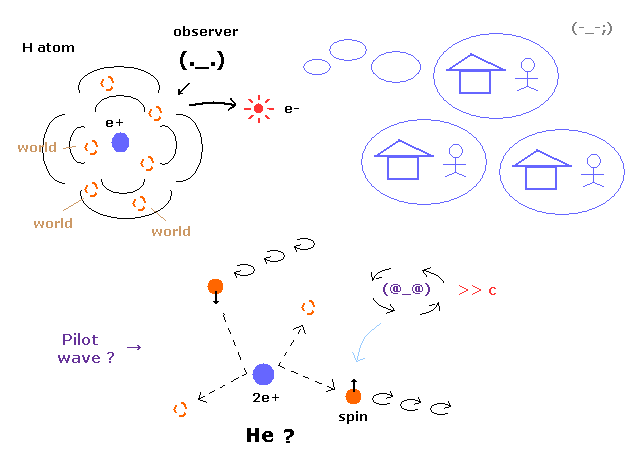As shown on this page, they couldn't decribe three-body helium by realistic model due to the lack of computer in 1920s.
For example, if you try to express helium using ( real ? ) pilot wave theory, two electrons always crash into nucleus ( l = 0 ), and chaotic, which gives extremely unstable helium. So this theory is completely inconsistent with actual helium.

So all they could do was accept unrealistic idea that wavefunction is just mathematical tool of probability.
And they just do "Shut up and calculate !" about interpretation (= Copenhagen ).
If they try to explain this wavefunction collapse using "local" theory, you must accept unrealistic many worlds.

So if standard model is correct, these completely meaningless debates about interpretation would continue forever, and make NO progress at all.

## Standard model needs many kinds of unrealistic "ether".

(Fig.4) Ether was denied → virtual particles, dark matter, energy, Higgs.. = ether ??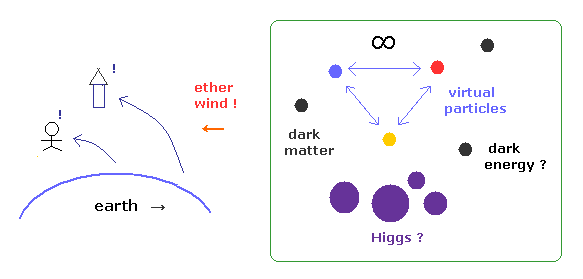Though they rejected "ether", relativistic quantum field theories accept infinite virtual particles, dark matter, dark energy and Higgs filling all the vacuum.

This idea is completely inconsistent with special relativity, which denied "ether".
In fact, the kinds of "ether" have been increased much more in relativisitic quantum field theory !

And as shown on this page, special realtivity includes fatal paradoxes (= Ehrenfest, trouton-noble, right-angle lever.. )
If they admit standard model is right, it means they continue hiding these fatal paradoxes from textbooks.
As a result, more new students and ordinary people with ambitions would be deceived, and have their life plans go awry.

## Experimental proof of quark is real ?

### [ Fractinal 1/3 charged quarks are really confirmed by experiments ? ]

(Fig.5) 1/3 quarks can NEVER be isolated → "quark" is just an illusion !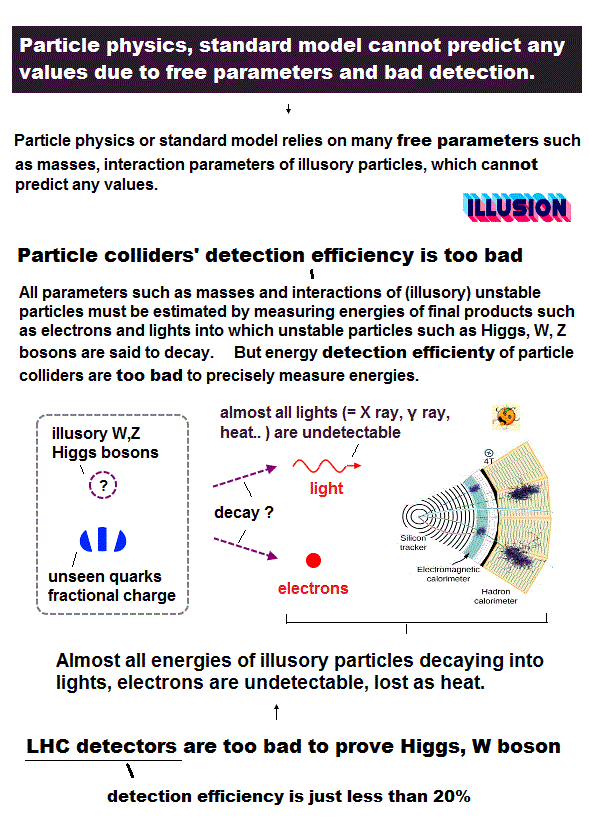Fractional charges (= 2/3e, -1/3e ) have NOT been observed, because quarks and gluons can NEVER be isolated.
Then, why they insist quarks have been actually found ? This is very strange.

There are too many elementary particles in the present standard model.
( So the word of "elementary" is very unreasonable, I think. )

There are three generations in quarks (= 2 × 3 = 6 ) and leptons (= 2 × 3 = 6 ).
Surprisingly top quark is as heavy as gold atom, though it is elementary particle with 2/3e charge !
Considering only the first generation stably exists inside proton and neutron, we can naturally think that unstable and useless second and third generations are only illusions inside accelerators.

## Inelastic scattering did NOT prove fantasy quarks.

(Fig.6) Inelastic scattering of electron and proton (= quark ? ).They insist deep inelastic scattering of high energy electron and proton showed the existence of quarks.
But unfortunately, these experiments could NOT show 2/3e or -1/3e fractional charges at all, as I explain here.

We always rely on Feynman diagram to express all phenomena in particle physics.
But as shown in this section, Feynman diagram always includes virtual particles, which contradict special relativity.

In Fig.6, an electron (= e- ) is scatterd by proton (= P ) by Coulomb force.
They insist in this process, the electron emits virtual photon (= q ) towards quark (= Q ) inside proton.

## Quark's momentum inside nucleus ?

(Fig.7) Quark (= Q ) has "x" times momentum of proton.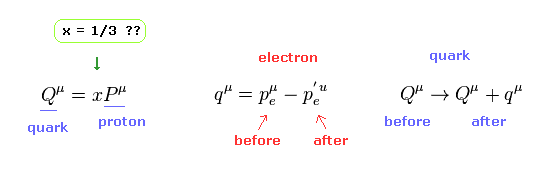We suppose each quark carry x times proton's momentum (= energy ).
If this "x" is 1/3, each quark's momentum is 1/3 × the whole proton's momentum.
Fig.7 is four-momentum of quarks (= Q ), electron ( p, p' = before and after scattering ), virtual photon (= q ).

These particles must satisfy energy-momentum conservation.
So the energy "q" (= photon ) is emitted from an electron, and then is absorbed into quark (= Q ).

(Fig.8) Einstein energy momentum relation.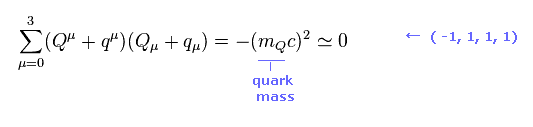And as shown on this page, each particle satisfies Einstein energy momentum equation.
mQ means the mass of each quark.

Here we neglect quark's small mass ( mQ = 0 ), so Fig.8 becomes

(Fig.9)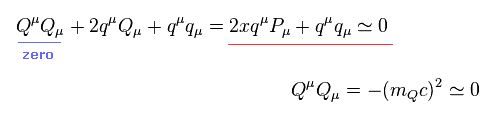Here Qμ = xPμ of Fig.7 is used.
As a result,

(Fig.10)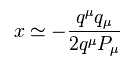Fig.10 is Lorentz scalar, so we can choose rest frame with respect to proton (= P ), keeping computing value invariant.

(Fig.11)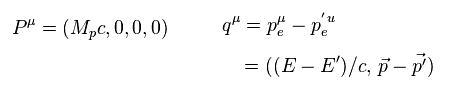Substituting Fig.11 into Fig.10, we have
( The mass of high energy electron can be considered to be zero. )

(Fig.12)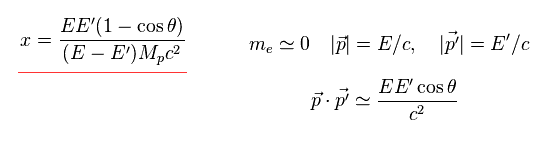From Fig.12, we can know the value of "x", when we measure energies (= E, E' ) of electrons, proton's mass (= Mp ), and scattering angle (= θ )
The experimental results of electron's distribution with respect to "x" become

## Experimental results did NOT show 1/3 quarks.

(Fig.13) Each quark is NOT 1/3 of proton.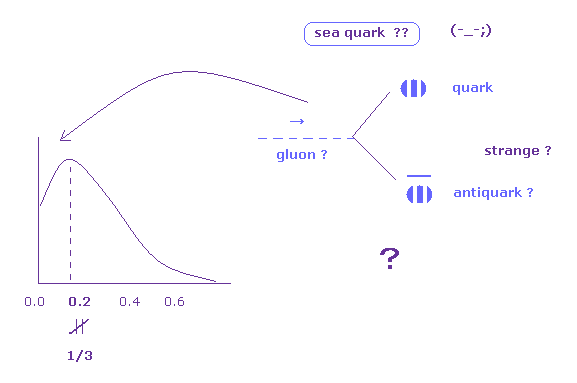As shown in Fig.13, each quark's energy ( momentum ) is less than 1/3 of the whole proton.
So these scattering experiments cannot show -1/3e or 2/3e fractional charges at all.

They introduced very artificial "sea quarks" inside proton to solve this problem.
"Sea quark" means gluons inside proton generate new pairs of quark and antiquarks, which have smaller momentum.
And due to these sea quarks, each quark's momentum becomes less than 1/3.
But of course, this sea quark can NOT be observed directly. These are only speculations and unrealistic.

## There is NO proof that "Jets" in the accelerator means quarks.

(Fig.14) "Color" string of quarks snaps. → new ( fantasied ) quarks ?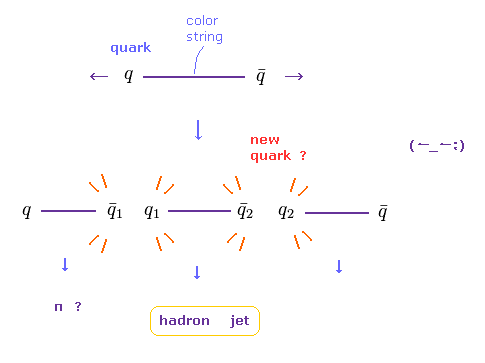Quarks with fractional charge can NEVER be isolated.
They insist "jets" (= collections of particle tracks ) mean unreal quark's generation.
Of course, this idea is only speculation, and if jet doesn't mean quark, standard model whould collapse at this point.

According to QCD ( quantum chromodynamics ), even if color strings binding two quakrs snap, new pairs of quark and antiquark (= fantasy ) are generated, which become hadron jets, they insist.

So a single quark is NEVER isolated. Do you think this theory is too good to be true ?
Of course, as hadrons such as π mesons are very unstable, we only estimate their existences from final products such as electron ( proton ), and photon.
( Very important "neutrino" cannot be observed in the accelerator ! )

## 2 jets = quarks,   3 jets = gluon.   Really ?

(Fig.15) Experimental proof of quark and "gluon" ? or they are just fiction ?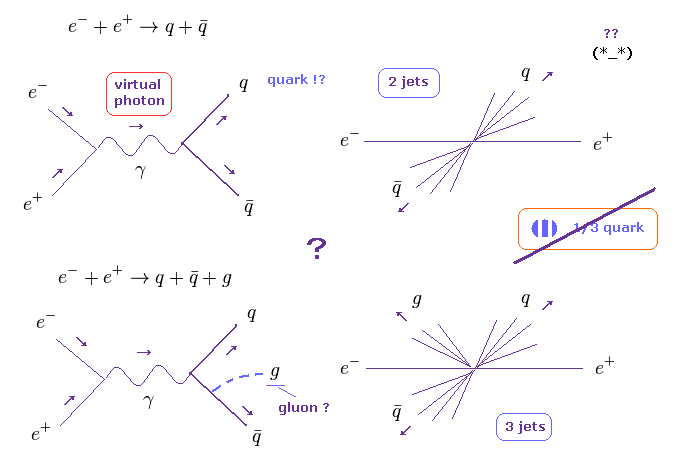In Fig.15 upper, a pair of electron and positron changes into virtual photon (= γ ), and then a pair of quark and antiquark is created (= two jet ).
Of course, this interpretation is just speculation, and virtual photon contradicts special relativity ( See this section ).

When three jets are observed, they insist one of them is the proof of "gluon".
Based only on this imaginary and speculative idea, they try to define coupling constant (= gs ) of quark and gluon.
( So this binding constant of strong force is free parameter, NOT standard model prediction. )

## Fantasy "spin" is dominant even in high energy accelerator.

(Fig.16) Pauli exclusion principle = "God" ?   Spin = "God" ?   absolute power !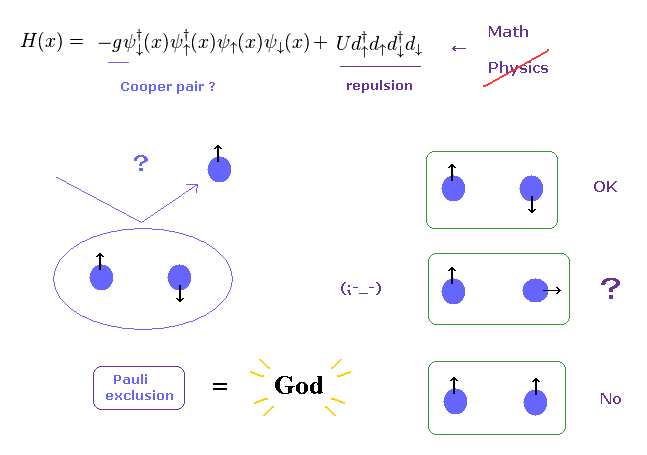In accelerator, they try to measure the kinds of "spin" ( 0, 1, or 1/2 ).
Of course, unreal spin is not real spinning, and cannot be observed directly.

They just estimate these spins, only from decay deviation of final products.
( In photon case, spin 1 is polarization of classical light. )

But this idea is completely unrealistic and unreasonable.
As shown on this page, spin magnetic moment (= fine structure level ) is too weak, so they cannot influence any reactions in the high energy accelerator at all, from the realistic viewpoint.
So under extremely high energy condition, very weak spin magnetic moment is completely meaningless and negligible.

Then why they loves this "spin" so much ?
The main reason is that they blindly believe and treat Pauli exclusion principle as "God" with absolute power.

## "Math" creation ( annihilation ) operators with "spin" obstruct Science.

(Fig.17) How "mathematical" operator can describe various phenomena, rich in variety ??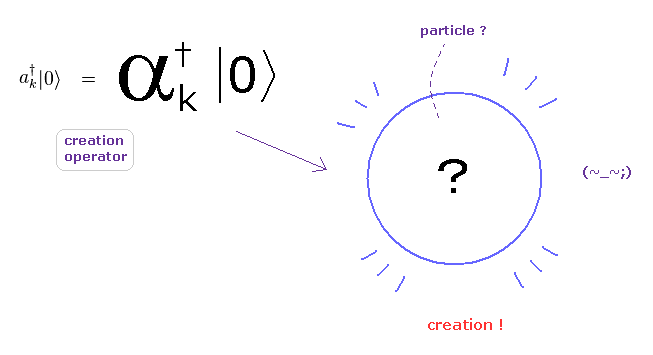Due to unrealistic spin and absolute Pauli exclusion , they must always rely on abstract math operators with spin.
In the present quantum field theories including condensed matter physics, standard model, and string theory, they completely rely on "mathematical" ( NOT physical ) operators to describe various phenomena.

As you see this page, these creation and annihilation operators do NOT have the power to express various dynamic and complicated phenomena in the actual world.
Because, when a particle is created ( or annihilated ), these operators cannot designate various differernt places and times in each different dynamic particle.

## Pauli exclusion principle remains "unsolved" → Abstract "math".

So the main obstructions to the development of sicence are these very abstract oparators caused by unreal "spin ".
"Photon", imaginary particle, needed to be introduced to agree with these creation (annihilation) operators.

Top journals such as "Nature" and "Science" should clarify this mysterious power of Pauli exclusion principle as soon as possible. ( NOT using abstract math symbols. )

## Scattering cross section really indicates quark's charge ?

(Fig.18) Total cross section ( σ ) = How large target's "area" is.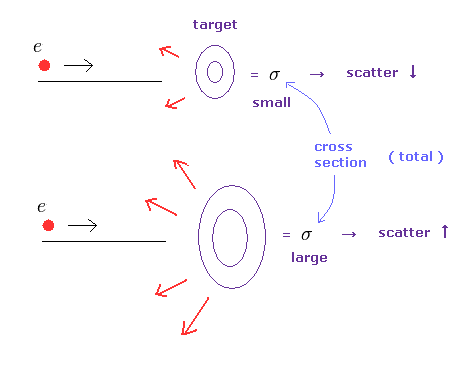Scattering cross section means the size of target's area.
So as this cross section becomes bigger, the target becomes larger, which means more scattering is observed.

(Fig.19) Scattering cross section ( σ ) is related to "charge". NOT quark's charge !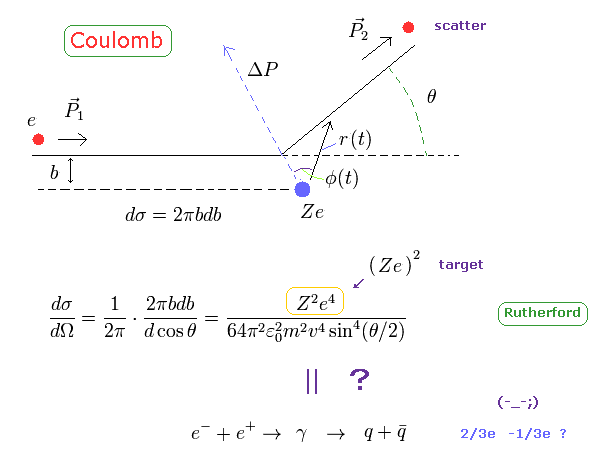As shown on this page, in classical Rutherford Coulomb scattering, cross section is in proportion to each charge.
In Fig.19, an electron (= e ) is scatterd by positive nucleus (= Ze ).
So charges of each particle ( before and after scattering, 2 × 2 = 4 ) is related to this cross section.
( Differential cross section (= dσ) means each infinitesimal cross section with respect to scattering angle, θ. )

This classical Rutherford scattering is Coulomb scattering.
So it is quite natural that we think each charge influences this scattering ( angle ).
The problem is whether each ( quark's ) charge is really involved even in the process of e- + e+virtual photon → quark, antiquark creation.

## Coupling constant in Feynman diagram gives quark's charge !?

(Fig.20) Lorentz force → Feynman diagram → "charge" = scattering ?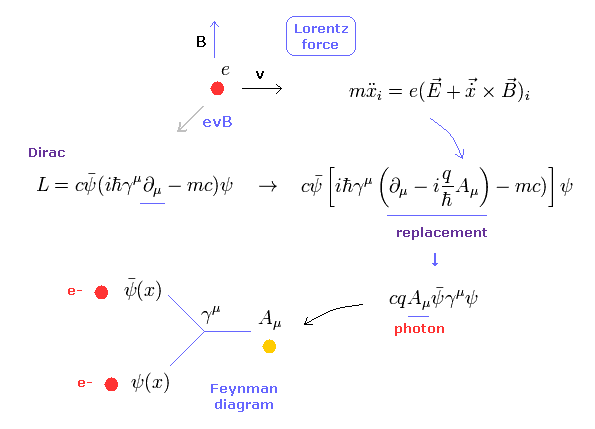As shown on this page, QED interaction among electrons and photon originates in classical Lorentz force.
In QED, a photon can be expressed as magnetic and electric potentials (= A, φ ).
If we do the replacements in Dirac's Lagrangian, we get the inteaction term, which gives Feynman diagram.

And this form of interaction is ONLY one interaction among electrons ( positron ) and photon in QED, though it is very abstract "math" symbols.
So they try to use this interaction term even in quark and antiquark creation.
As a result, binding constant charge "q" (= Ze ) is related to all kinds of scattering amplitude, they insist.

## Coulomb scattering and annihilation are completely different.

(Fig.21) But electron-positron annihilation is completely different from Coulomb scattering !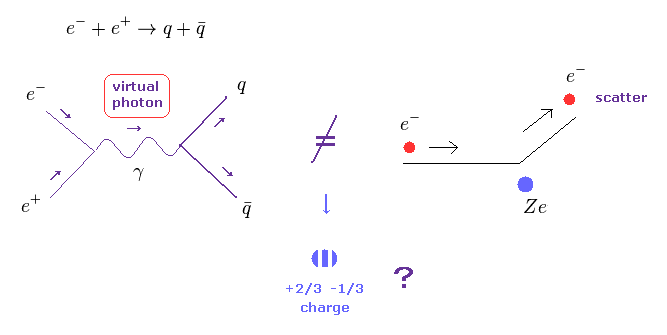As you see Fig.21, electron-positron annihilation is completely different from usual Coulomb scattering.
Because, in usual Coulomb scattering ( ex. an electron is scattered by proton ), each charged particle does NOT disappear at all, they are just scatterd by each other.

But in Fig.21 left, electron and positron are annihilated into virtual photon, and then new quark-antiquark are generated !
So these processes are completely different kinds of things.
The idea that "imaginary" new quark's charge is involved in this creation process is very unreasonable and unrealistic.

## Experimental proof of fractional charges are very doubtful.

(Fig.22) Experimental proof of fractional charge ??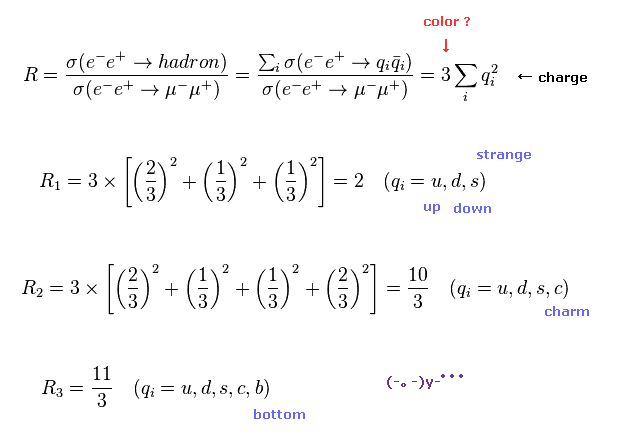So they insist total cross section is proportional to a square of each charge of new generated quarks.
( Though each quark can NEVER be isolated. )
Each quark has three colors (= red, green blue ), so they suppose these three colors are generated just equally.
( Of course, these cannot directly be confirmed, these definitions are only speculation. )

Furthermore, they suppose different kinds of quarks (= up, down, strange, charm, bottom ) are generated at the completely same probability !
Though their mass energies are completely different ( bottom quark is more than 1000 times heavier than up quark ! )

## Very far-fetched interpretation about quarks.

(Fig.23) Expetimental proof of fractional charge ??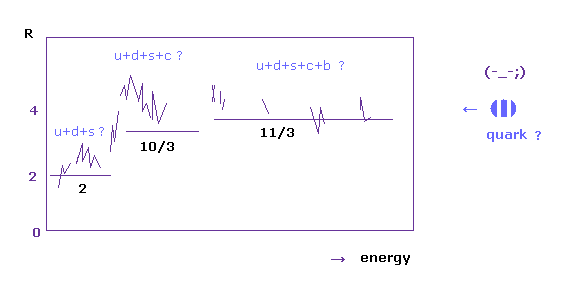Fig.23 is the ratio of quark's generation to muon generation, they insist.
As you see in Fig.23, scattering data are very vague (= NOT determined as a single constant ).
So the interpretation of Fig.22 is very artificial.

There is NO guarantee that each jet really means quark.
So quark existence is completely dependent on "ad hoc" standard model theory.

## "Same" probability of producing "tiny" up quark and "massive" bottom quark ?

(Fig.24) Probability of "up", "down", "bottom" quarks are the same ??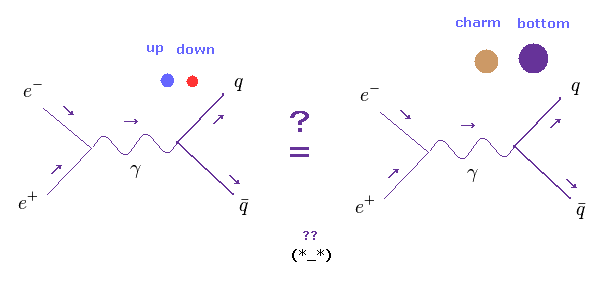As you know, the numbers of very light atoms such as hydrogen and helium are much larger than any other heavy atoms in the universe.
So it is quite natural that light particles (= up, down quarks ) are more easily created than extremely heavier quarks (= charm, bottom ).
As a result, the interpretation of Fig.22 is very unnatural.

## Asymptotic freedom of quarks was NOT confirmed.

(Fig.25) Expetimental proof of asymptotic freedom ??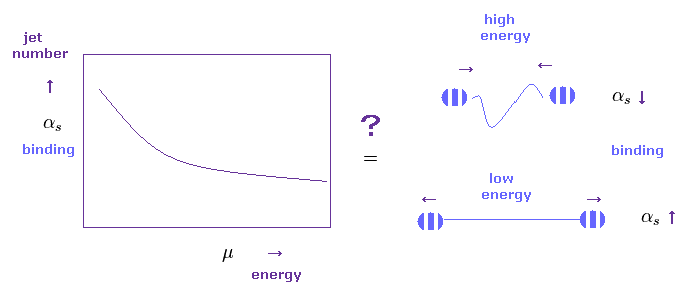They insist the power of color string between quarks becomes weaker, as the distance between quarks is shorter (= high energy ).
On the other hand, as this distance becomes longer (= low energy ), binding power of this string becomes stronger.
( Of course, these ideas are only speculative, and just fantasy. )

As binding constant becomes bigger, scattering ( jet ) number becomes bigger, according to Feynman diagram interaction.
So, they insist the scattering data of Fig.25 proved this asymptotic freedom.
But unfortunately, we cannot prove this strange property only from these indirect data.

## Coupling constant of strong force is very artificial.

(Fig.26) Coupling constant of strong force ?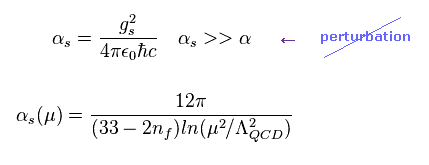They suppose coupling constant (= strength ) of strong force changes like Fig.26.
Of course, this constant gs is one of free parameters, which cannot be predicted from standard model itself.
In Fig.26, as the energy (= μ ) becomes bigger, binding strength (= α ) becomes weaker.

Due to this strange property of asymptotic freedom, coupling constant becomes too strong in low energy area.
So quantum chromodynamics cannot use perturbation of QED and cannot predict various values.

## Natural interpretation of experimental results.

(Fig.27) Natural interpretation of Fig.25 data.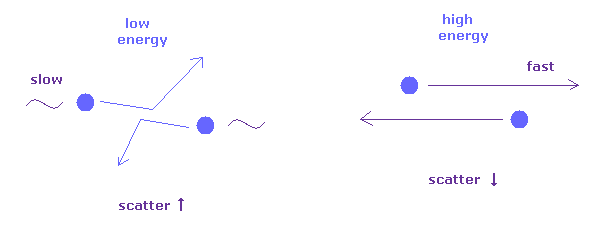It is natural that we think, under the condition of extremely high energy, each particle with high speed tends to easily pass each other, not scattered.
Under the lower energy, interaction among them becomes dominant (= not negligible ), while they are passing each other.
This interpretation is more natural and realistic than imaginary quarks and strange freedom.

If you insist this color string is true, what is the origin of string, and how this color string is made ?
Don't escape just saying "Shut up and calculate !" like various journals.

## Experimental proof of W, Z bosons is real ?

### [ Heavy W boson violates energy conservation → "unreal" particle ! ]

(Fig.28) Very heavy W boson (= 80 times proton ) is real ?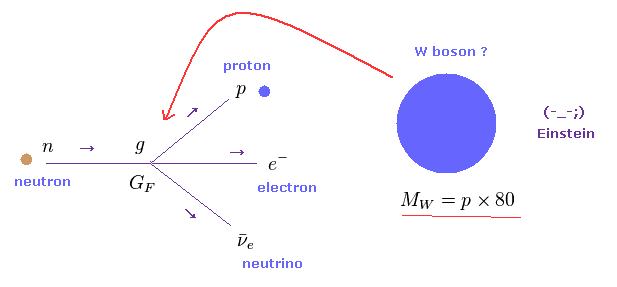In beta decay, neutron changes into proton, emitting electron and antineutrino.
The mass energy difference between neutron and proton is very small, so it is a very unreasonable idea that very heavy W boson (= 80 times proton ! ) is created in this process.

This process completely violates energy conservation.
So they try to use very vague and "ad hoc" concept of time-energy uncertainty principle to explain this large discrepancy.
Can you believe this unrealistic standard model's interpretation ?

## Coupling constant of weak force.

(Fig.29) Coupling constant of weak force ?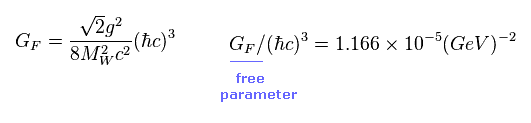GF is a free parameter involved in weak force binding.
This value cannot be predicted from standard model itself, it is estimated from life time of beta decay.

## Weinberg angle θ links W and Z bosons ?

(Fig.30) W boson mass ?Coupling constant "g" is also free parameter, which cannot be predicted from standard model itself.
As shown on this page, this "g" is expressed using Weinberg angle θW.
This angle is also a free parameter.
( When one of them is determined, another is determined. )

From Fig.29 and Fig.30, W boson mass is determined.
Of course, we cannot know whether MW inside GF of Fig.29 really means W boson mass.
As I said, if this W boson mass is real, law of energy conservation is completely broken.

(Fig.31) Z boson mass ?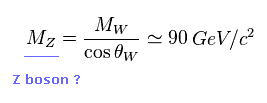If angle θW is determined by some experiment, Z boson mass is also determined.
Of course, "Z boson" itself is an artificial neutral particle created for unifying electroweak force.
The moment they define sin, cos θW, this new Z particle needs to be created, too..

## Very heavy W boson is inconsistent with the energy of β decay.

(Fig.32) β - decay. ( neutron → proton + electron + antineutrino. )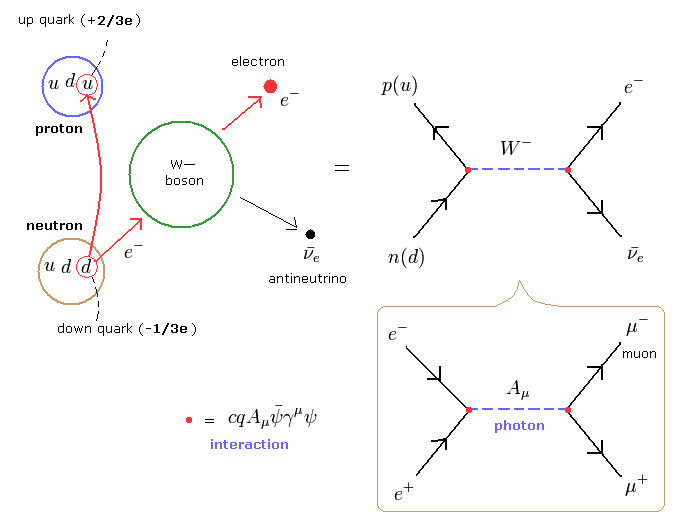All reaction processes must obey Feynman diagram.
In this process, down quark inside neutron emits heavy W boson, and changes into up quark inside proton.
And this W boson changes into electron and antineutrino, they insist.

As shown in this section, this W boson (= middle line ) is virtual particles, which disobey special relativity.
So standard model includes self-contradiction from the beginning.

## Neutrino = "missing" energy is one of tricks.

(Fig.33) W boson decay modes.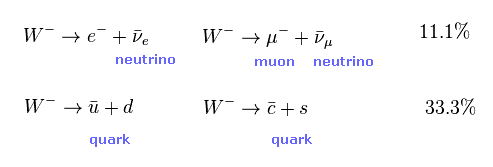W boson decays more often into quarks than electron and neutrino, they insist.
But they didn't use the quark decay mode (= lower line of Fgi.33 ).
Because, quarks and gluons may be scatterd by strong force, which process is random and unpredictable.
And the probability of this random scattering by strong force is bigger than the probability of W, Z boson creation.

## It's impossible to know correct "missing" energy.

(Fig.34) Experimental proof of W boson ?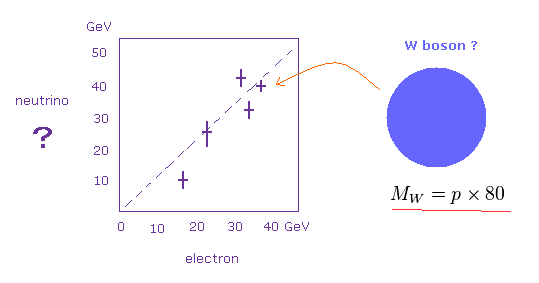The important point is that we cannot measure neutrino, which can pass through even the earth easily.
To know the number of this generated neutrino, they need to measure all new particles possibly generated ( impossible ! )
And if there are some energy bias (= loss ), it means generation of unseen neutrino, they insist.

But this judgement is only speculative and unreasonable .
Because there are infinite irrelevant noises in comparison to very rare W boson generation.
So this estimation of unseen neutrino is very doubtful .

As shown in Fig.34, in about 80 GeV site (= electron + unseen neutrino ), some resonant points appear.
( But there are other resonant points, which is not 80 GeV. )

## Z boson cannot be observed directly, either.

(Fig.35) Z boson decay modes.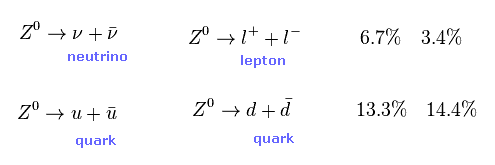Also in case of Z boson, they estimate Z boson mass from scattering events (= cross section ) of final products.
So unstable W and Z bosons cannot be observed directly.
( These very unstable particles cannot be seen even in cloud chamber. )

## Rare W boson in the accelerator is illusion, NOT actual partcle.

(Fig.36) W boson in the accelerators is NOT equal to the beta decay.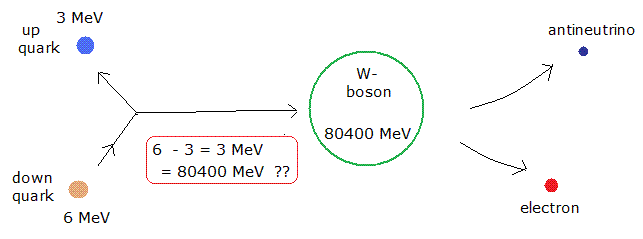The ratio of W and Z bosons are predicted by the relation of Fig.31.
So to agree with this relation, they tried to find some resonant points (= free parameter ).
This resonant points ( or Weinberg angle θW ) is free parameter, which cannot be predicted by standard model.

As a result, this resonant point gives unrealistically heavy W boson, which violates energy consrvation like Fig.36.
Do you think this very heavy W boson, which appears ONLY in extremely high energy accelerator, is really involved in usual beta decay ??   ( I don't think so. )
To fit standard model theory, all they can do is accept this unrealistic heavy W boson. This is the truth.

## Time - energy uncertainty principle gives particle's lifetime ?

(Fig.37) Mean life time and decay width = uncertainty principle is real ?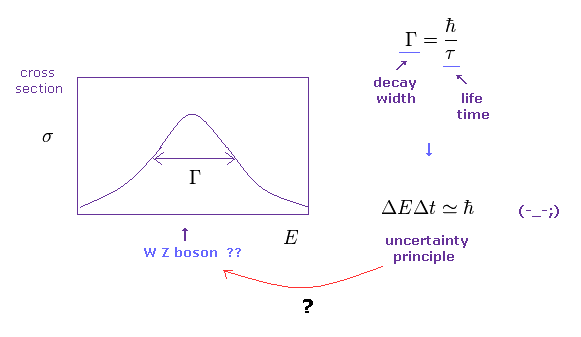The interpretation of events in high energy accelerator, they completely depend on time energy uncertainty principle.
So if this presupposition is wrong, all interpretation of the present standard model is wrong, too.

As energy (= mass ) uncertainty (= width ) of Fig.37 becomes wider, uncertainty of time becomes smaller (= life time is shorter ), they insist.
So they insist line width means decay width, which means how easily particle decay.
Of course, these interpretation is only speculation, has NO grounds at all.

Fig.37 data only shows some scattering event distribution, and does NOT mean new particle creation ( and life time ) at all, I think.

## Poof of three generation of neutrino is very doubtful.

(Fig.38) Experimental proof of "three" generations of neutrino ??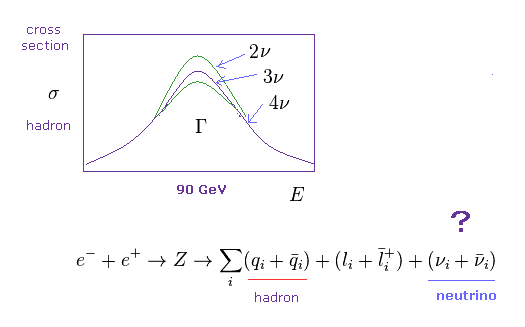As I said above, unseen neutrino cannot be observed directly, though they are very important in all reactions.
They insist decay width of Fig.37 means the total decay probabitliy including even these unseen neutrino.
( Of course, this assumption is a completely "imaginary" thing. )

So if we measure all scattering of other hadrons, they can estimate the decay probability of remaining neutrino.
When this difference is big, this means the number of ( unseen ) neutrino generation is big.
From this speculative assumption, they conclude this experiment (= Z boson decay ) can show neutrino has three generations, and standard model's prediction is right !

But in fact, the recent results of LSND and MiniBooNE show the possibility of fourth new generation of neutrino.
And first, neutrino oscillaltion (= neutrino mass ) completely contradicts standard model.
Even when they get the results inconsistent with the conventional standard model, they NEVER try to reject standard model.
( They use very convenient phrases such as "beyond" standard model at these times. )

## 90 × protons = Z boson can often appear in real world ?

(Fig.39) Experimental proof of neutral Z boson ??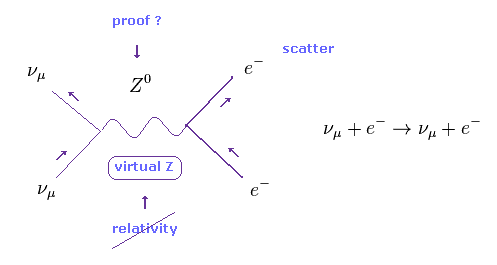When they find some neutrino influences electron's motion, they conclude that this phenomenon proves the existence of Z boson.
But of course, they didn't see very heavy Z boson itself.
We can naturally think, in this phenomenon, de Broglie wave (= neutirno ) scatters an electron a little.

So unrealistic Z boson is completely unnecessary.
And do you think Z boson as heavy as 90 times proton is really generated in this process ?

## Origin of mass and SUSY.

### [ 98 % of nuclear mass is caused by "chiral symmetry breaking", Higgs is only 2 % ? ]

(Fig.40) Chiral symmetry breaking can explain 98 % of nuclear mass.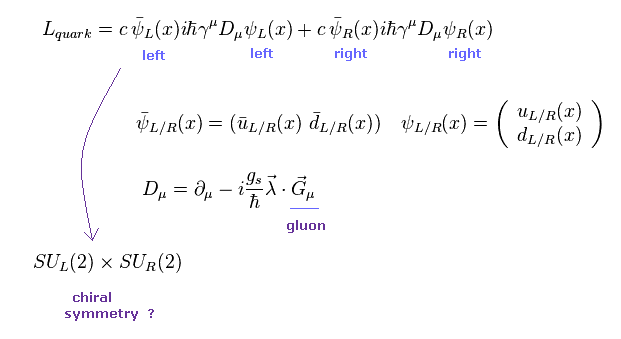It is said that 98 % of nuclear mass is generated by chiral symmetry breaking, and only 2 % of mass is caused by Higgs mechanism.
Then what is chiral symmetry ?
Unfortunately, there are NO concrete physical images in this chiral symmetry (= QCD ), as shown in Fig.40.
( These are only abstract "math" symbols. )

In Fig.40, they approximately suppose quark's mass is zero, so they can separate left-handed (= L ) and right-handed (= R ) of quarks.
Like other forces, this Lagrangian has some symmetry, it means it is invariant under phase transformation in L and R independently.
This is called "chiral symmetry" ( SUL(2) × SUR(2),   SU(2) means 2 × 2 matrices ).

## Chiral symmetry breaking is just math, NOT physics.

(Fig.41) Symmetry breaking = quark-gluon aggregation ??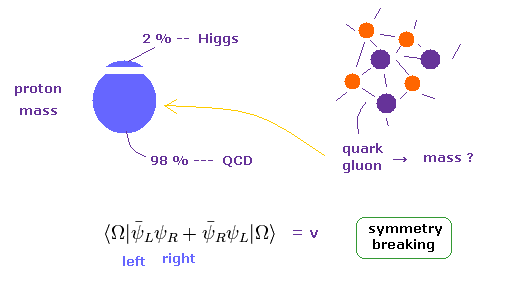When the vacuum includes mixed L and R fields, it is NOT invariant under phase transformation (= chiral symmetry breaking ).
In this situation, they insist many pairs of quark and antiquarks fill the space inside tiny proton !
This mechanism of QCD can explain 98 % mass of proton, they insist.

This strong force cannot use usual perturbation methods such as QED due to strong coupling constant.
So all they can do is estimate their behavior only from some approximate numerical calculation.
Unfortunately, there are NO realistic grounds in this theory at all.

## Why they need unrealistic supersymmetry (= SUSY) ?

(Fig.42) Grand unification theory (= GUT )??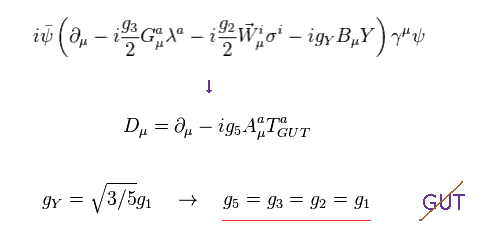In fact, the present theory cannot completely unify all three fundamental forces such as electromagnetic, weak and strong forces, besides gravity.
To unify all these forces, three coupling constants become the same in some extremely high energy area.

SUSY-GUT (= GUT + supersymmetry ) can solve this problem by new parameters.

## Hierarchy problem of Higgs mass is NOT physics.

(Fig.43) Hierarchy problem of Higgs mass ?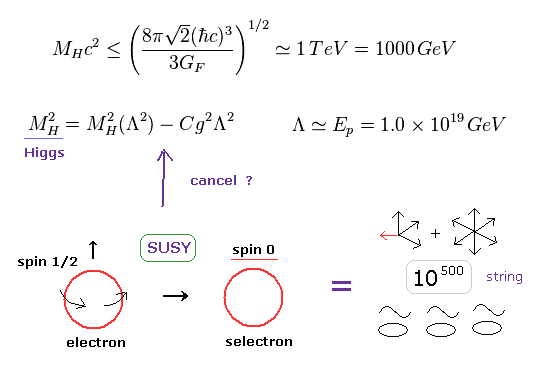The present standard model cannot predict Higgs mass at all.
Furthermore, when this Higgs mass exceeds some critical point (= 1 TeV ), the standard model breaks down.
( So the result of 126 GeV Higgs is within this area. )

According to self-interaction of Higgs-quark-gauge particles, Higgs mass diverges to planck energy level (= 1019 GeV ).
To get realistic Higgs mass (= 126 GeV ), this correction needs to be cancelled out by the original Higgs mass (= planck energy level, too ).
Supersymmetry is the ONLY method to do this cancellation. So SUSY is indispensable for this mathematical ( NOT physical ) reason.

As you feel, this cancellation of almost infinity - infinity is very unrealistic and unnatural.
Furthermore, supersymmetry is indispensable for changing 26 dimensions to 10 dimensions in string theory.
( Though both dimensions are unrealistic, as you see. )

## Virtual particles contradict special relativity.

### [ Scattering in particle physics contradicts special relativity ! ]

(Ap-1) Coulomb scattering → virtual photon → Einstein is wrong ?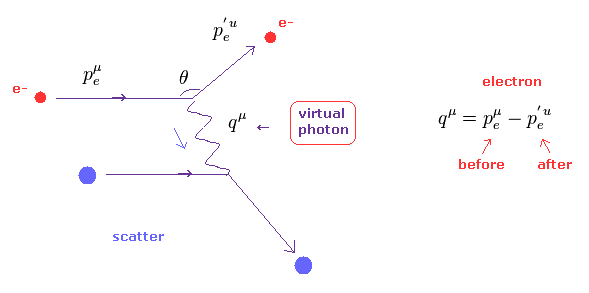As you know, relativistic quantum field theories such as QED, standard model and string theory must satisfy special relativity.
But in fact, all these relativistic theories violate special relativity in all reactions.

Ap-1 shows Feynman diagram of electron's scattering (= e-, red ) by some charged particle (= blue ).
In this process, they insist electron exchanges some "photon" between the target particle.
This photon is called "virtual photon", because it does NOT satisfy special relativity.

(Ap-2) Four-momentum before and after scattering.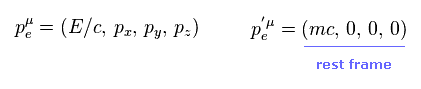Here we think about the rest frame with respect to electron after scattering (= p' ).
"E" means relativistic energy, and "p" means each component of electron's momentum.

(Ap-3) Relation of four-momentum.It is known that these four momentums satisfy Ap-3 in all reference frame.

As you see Ap-3, this equation always becomes positive because a square of mass is positive.
But in case of virtual photon, this value always becomes negative (= tachyon ).
This fact clearly proves relativistic standard model includes self-contradiction.

(Ap-4) Energy conservation.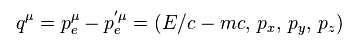In Ap-1, electron "p" emits photon q, and becomes p'.
So energy and each component of momentum must be conserved.
As a result, four momentum of photon q becomes Ap-4.

(Ap-5) Photon "q", Einstein's energy momentum equation.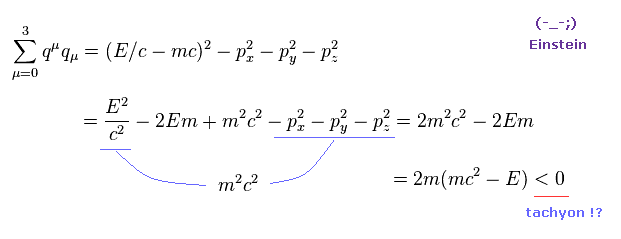Energy- momentum equation ( see Ap-3 ) of this photon q becomes like Ap-5.
Using Ap-3, we can prove the scalar mass of this photon q becomes negative.
This means this photon is "tachyon", which Einstein would NEVER admit.

qμqμ is scalar constant, so in all reference frames, this value always become negative.
The important point is that the present particle physics completely depends on this strange tachyon.
This means standard model is just wrong.

### [ "Electon - positron → photon" contradicts special relativity ! ]

(Ap-6) Eelectron - positron → virtual photon → quarks ?In the accelerators, annihilation of a pair of electron ( e- ) and positron ( e+ ) is often used to generate new particles such as quarks, W, and Z bosons.
But in fact, these processes always violate special relativity, which means these new particles are unreal.
( W, and Z particles themselves are unreal virtual particles. )

In Ap-6, electron and positron are annihilated, and changes into virtual photon (= γ ), and then becomes a pair of quark and antiquark.
( Of course, these quarks can NEVER be isolated, so these are only imaginary particles. )

(Ap-7) Energy and momentum in center-of-mass system.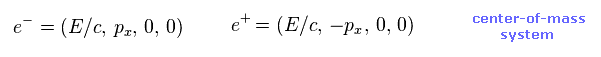Ap-7 is a simple example, in which electron and positron are just moving in the opposite directions and crash into each other ( see also Ap-6 right ).
In this center-of-mass system, energies E of them are completely the same, and momemtum's directions is just opposite.
( "px" and "-px" in electron and positron, respectively. )

(Ap-8) Energy and momentum of virtual photon γ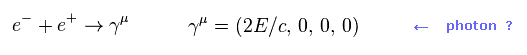As a reault, summing each component of Ap-7, we get photon's energy and momentum of Ap-8.
( Here "γ" doesn't mean γ matrices, it is γ rays. )
At a glance, you can find this photon of Ap-8 is NOT real photon.

(Ap-9) Because this photon's mass is NOT zero !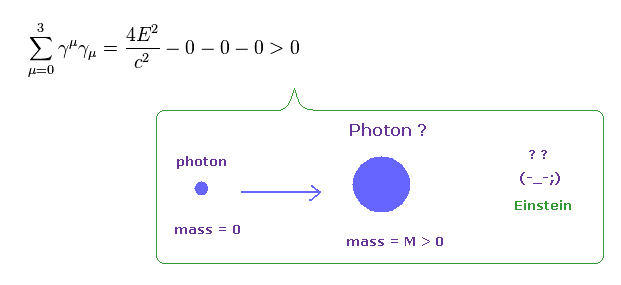Energy-momentum equation of this photon γ gives positive mass.
This photon with mass clearly contradicts special relativity.
So all new particles and processes in the present standard model are inconsistent with special realtivity.

Again, this fact proves the present stanard model (+QED, string theory ) is just wrong.

### [ Antiparticle are real ? ]

(Ap-10) Positron is a real particle or other things ??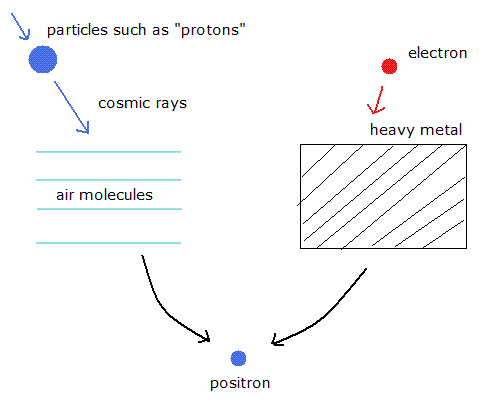Positron was found by Anderson in 1936.
But even after these experiments, Millikan, who was his teacher, and Dirac doubted its existence.
Actually inside the cloud chamber, very few positron-like particles could be found.

Positron is thought to appear from vacuum according to the quantum theory.
But actually, when we try to make positron, we have to hit the electrons into things such as heavy metals ( NOT vacuum ).
And the positron in the cosmic rays is produced when particles such as protons crash into the air molecules ( NOT vacuum ).

(Ap-11) Why heavy "nucleus" is indispensable for antiparticle ?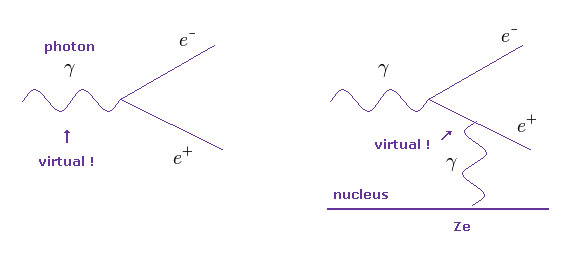As shown in Ap-11 left and Ap-6, if there is no nucleus, this photon generating a pair of electron and positron is virtual photon, which violates special relativity. See also this page.

On the other hand, if some nuclei are involved in this process, virtual particles are hidden in the middle lines (= Ap-11 right ).
So they insist, some heavy metal's nuclei are indispensable for generating a pair of electron ans positron.

But as you feel, the existences of these unreasonable virtual particles themselves mean these reactions are NOT real, irrelevant to middle or external lines.

(Ap-12) Which will happen ?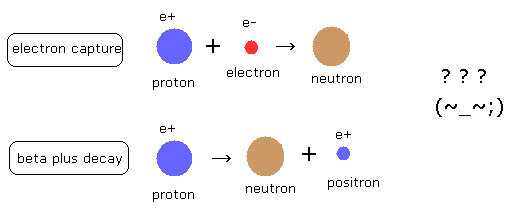The beta plus ( β + ) decay and the electron capture generate the same nucleus.
Both of them change one proton in the nucleus into a neutron.
Of course, this reaction happens only when the final product is energetically favorable in the nuclei.
As a result, both reactions emit energy.

But as you know, neutron is a little heavier than proton, so beta plus decay clearly contradicts energy-mass conservation.
They insist we need to consider interactions with other particles, but Feynman diagram clearly admits this single independent reaction.
This is strange.

(Ap-13) "Positron" is contradicory. "Where" is the energy emitted ?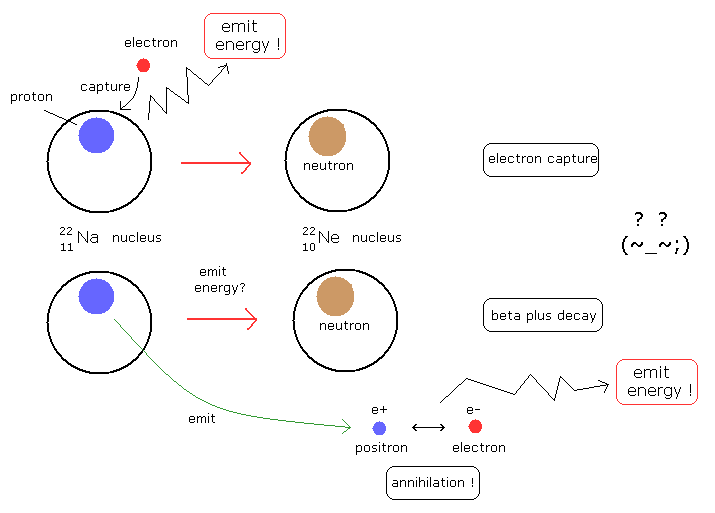In Na (22 11), both the electron capture and beta plus decay can occur.
In the electron capture, when the nucleus changes into the stable one capturing one electron, it emits energy.
But in the beta plus decay, when the nucleus changes into the stable one, it does NOT emit energy but emit a positron.
And then the positron meets a electron somewhere else, annihilating each other, and emit energy.
( This means one of the nuclear energy is "teleported" into the external electron ONLY in beta-plus decay ? )
So there is a self-contradiction in the places where the energy is emitted as the same nucleus is generated.
This means beta plus decay (= positron ) is contradictory, and it is just electron capture.

For example, it is said that in proton-rich nuclei where the energy difference between initial and final states is less than 2mec2, then β+ decay is not energetically possible, and electron capture is the sole decay mode.
This means these reactions are basically the same things ?
( As the positron and electron have some momentums, the annihilation energy is usually greater than 2mec2. )
Of course, these nuclei can NOT predict the electron mass energy flying from somewhere else, when they emit the positron.

(Ap-14) Positive potential energy (= mc2 ) means very unstable electron !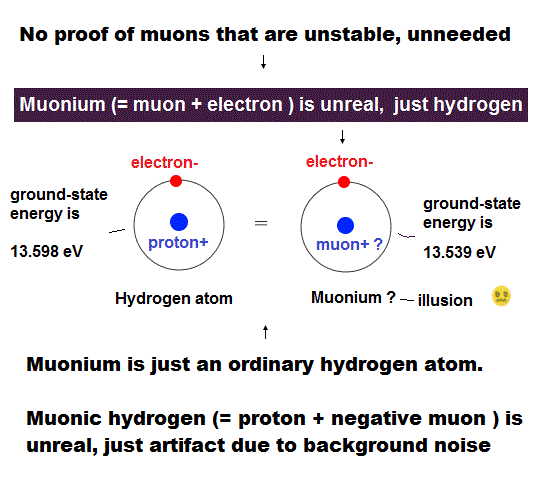As you know, an electron is one of most stable elementary particles.
But if a single electron's energy is positive like mc2, the electron is very unstable and easily broken.
Because it is natural that stable state means its potential energy is negative.
So the big positive potential energy of mc2 is very unnatural.

Enen if you consider some spherical walls which confine this big mass energy into very tiny electron, the total energy must be negative for stability.
For this spherical walls to withstand big positive mass energy inside, this spherical walls must be very stable and bound tightly to each other by bigger negative energy. ( So the total must be negative energy to be stable electron. )

### [ Positron inside cloud chamber is real ? ]

(Ap-15) Positron or proton ?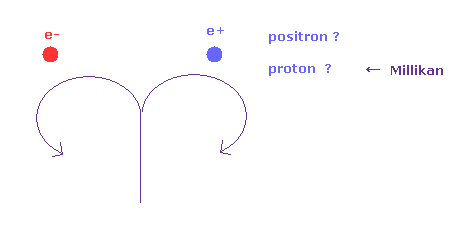Positive and negative charged particle rotate in the opposite directions in magnetic field B.
So they insist Ap-15 cases in the cloud chamber mean one is electron and another is positron.
But Robert Millikan NEVER admitted this positron throughout his life.
Millikan said this positron is just proton or something.

Even if proton and electron (positron) have the same momentum, proton easily stops, because proton has smaller kinetic energy due to its heavy mass, they insist
So they insist "proton" tracks inside cloud chamber must be shorter and thicker than positron.
But this story is just about the average behavior of the protons. ( NOT always. )
There are infinite protons inside cloud chamber, so some of them may fly longer than expected, especially when they have high energy.

The probability they crash into other particles are random.
And high energy muon (= electron ) and proton may NOT easily interact with other charged particles.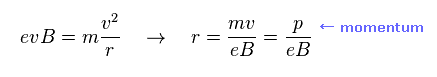As shown in Ap-16, the rotating radius of charged particle is determined by its momentum NOT by its mass.
( So useless particle "muon", which easily decays into electron, can be considered as a kind of high energy electron. )
And in the usual accelerator, we cannot distinguish positron and protons, and cannot measure their high speeds ( = "c" ).
They only estimate their existences by some shower patterns.

Different from alpha particle (= 2e+ ), proton has the same positive charge as positron.
And it is known that neutron, which is as big as proton, does NOT show any track in the cloud chamber.
So, it is too simple to conclude that proton's track always become bigger and shorter.
Though they have NEVER seen positron itself before, why they can distinguish the difference between high energy proton and positron ? It's impossible.

(Ap-17) How antiparticles distinguish and adhere to their particles ??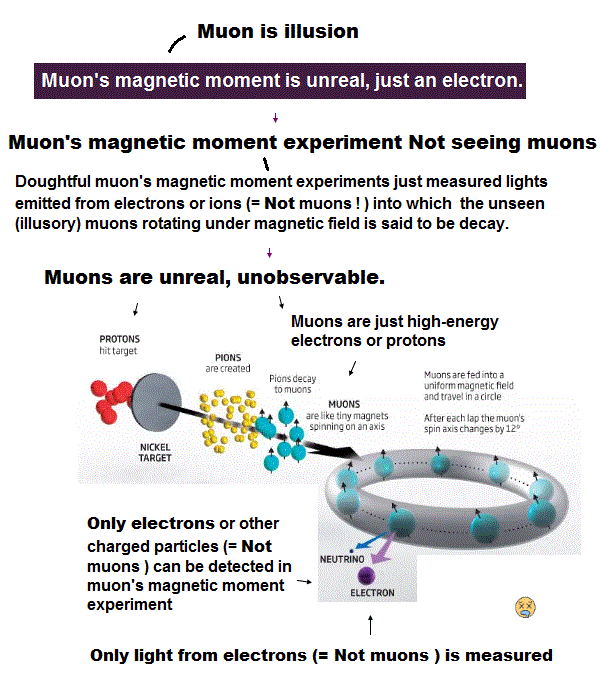In real science, the instant we know the leukocytes adhere to blood vessels, we naturally try to investigate what adhesion factors ( LFA, ICAM-1 ) are related.
But they NEVER try to investigate these adhesion factors between electron and positron ( and proton and antiproton ), though as much as 80 years have passed since positron was discoverd.

As shown on this page, unstable antiparticles can be naturally replaced by electron, proton, and neutron captures.
And it is unnatural that very stable electron's energy (= mc2 ) is not negative.
"Not asking" for very long term means they admit these concepts are only mathematical things.2013/10/8 updated. Feel free to link to this site.